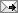Saturday, July 11, 2015:

# A ‘general formula’ for the price-rent ratio in a rational property market

How pro-speculation tax systems can lead to “sub-intrinsic-value bubbles”This page uses MathJax™ to display equations and mathematical symbols. It requires JavaScript™ and an up-to-date browser.

### The formula

In a rational property market, the gross rental yield of a property, i.e. the reciprocal of the price/rent ratio, is given by \begin{equation}\label{eygen} y = h + \frac{i-g/u}{1-e^{(g-ui)T}} \left\{ (1\!+\!s)\left[1\!-(1\!-\!v)e^{-uiT}\right] - (1\!-\!r)ve^{(g-ui)T\strut} \right\}\,, \end{equation} where

• $$s$$ is the stamp duty rate payable by the buyer on the sale price of the property (negative for a grant or subsidy);
• $$r$$ is the resale cost payable by the seller, expressed as a fraction of the resale price, and includes any commissions, legal fees and “vendor stamp duty”, but not capital-gains tax (which is treated separately);
• $$h$$ is the holding charge rate, expressed as a fraction of the current market price per unit time, and includes any property taxes, “rates”, maintenance costs and body-corporate fees;
• $$u$$ is the fraction of current property income and related expenses remaining after income tax;
• $$v$$ is the fraction of any net capital gain remaining after income tax;
• $$g$$ is the continuously compounding rental growth rate; thus the rent at time $$t$$ is \begin{equation}\label{eE} E = E_0\,e^{gt}\,, \end{equation} where $$E_0$$ is the initial rent (at $$t\!=\!0$$);
• $$i$$ is the continuously-compounding grossed-up discount rate; that is, $$ui$$ is the continuously-compounding after-tax discount rate, so that a future cash flow at time $$t$$ must be multiplied by \begin{equation}\label{edisc} e^{-uit} \end{equation} to find its present value (PV) at time $$0$$;
• $$T$$ is the time for which the property will be held;

and $$y$$, $$s$$, $$r$$, $$h$$, $$u$$, $$v$$, $$g$$ and $$i$$ are assumed to be constant through the holding period.

(N.B.: Defining $$i$$ as the grossed-up discount rate is convenient for interpreting certain special cases, but is not meant to imply that the grossed-up rate is more fundamental than the after-tax rate.)

### So what?

The formula explains the empirical observation that a stamp duty on property buyers can reduce prices by more than the value of the duty, while a subsidy (or “grant”) for buyers can raise prices by more than the value of the subsidy.

If rents are rising, the formula predicts absurdly high price/rent ratios for reasonable values of the parameters. Thus it predicts the existence of sub-intrinsic-value bubbles — situations in which prices, although more than justified by net-present-value calculations, are unsustainable due to unserviceable debt. It also indicates that tax reform involving greater reliance on land-value tax and/or capital-gains tax can prevent sub-intrinsic-value bubbles by bringing “intrinsic” values (net present values) within the debt-servicing capacity of prospective buyers. The capital-gains-tax option is found to be more effective in reducing the annual cost of home ownership relative to renting. As these conclusions say nothing about taxation of consumption or labour income, they have no bearing on the overall level of taxation or public expenditure.

### Derivation

By the above definitions, if $$P$$ is the market price at time $$t$$, then \begin{equation}\label{ey} E=yP\,. \end{equation} From $$(\ref{eE})$$ and $$(\ref{ey})$$ we have \begin{equation}\label{eP} P = E_0\,e^{gt}/y\,, \end{equation} showing that the rental growth rate $$g$$ is also (unsurprisingly) the price growth rate. The initial price (at $$t\!=\!0$$) is \begin{equation}\label{ePo} P_0 = E_0/y\,. \end{equation}

Let the property be bought at $$t\!=\!0$$ and sold at $$t\!=\!T$$.  Let $$P_0^{\rm\,rent}$$ denote the PV of the rent received during the holding period, and let $$P_0^{\rm\,resale}$$ denote the PV of the resale price, where both the rent and the resale price are net of taxes and other costs (but not interest, which is accounted for in the discount rate). For the initial buyer, the net present value (NPV) is $$P_0^{\rm\,rent}$$ plus $$P_0^{\rm\,resale}$$.

Before income tax, the rent net of holding charges is $$E\!-\!hP$$.  After income tax, the net rent received or saved during an infinitesimal interval $$dt$$ is \begin{equation}\label{erdt} u(E-hP)\,dt\,, \end{equation} which we multiply by $$e^{-uit}$$ to obtain its present value. Adding the PVs for all the infinitesimal intervals, we have \begin{equation}\label{ePrent} P_0^{\rm\,rent} = \!\int_0^T \!\! u(E-hP)\,e^{-uit}dt\,. \end{equation} Substituting from $$(\ref{eE})$$ and $$(\ref{eP})$$, we find \begin{align} P_0^{\rm\,rent} &= uE_{0\,}(1-h/y)\!\int_0^T \!\! e^{(g-ui)t}dt\label{ePrentTi}\\ &= \tfrac{E_0(1-h/y)}{i-g/u}\left[1-e^{(g-ui)T}\right]\label{ePrentT} \end{align} provided that $$g\!-\!ui \neq 0$$.

The acquisition price including duty is $$P_0(1\!+\!s)$$, which, by $$(\ref{ePo})$$, can be written \begin{equation}\label{ePos} E_0(1\!+\!s)/y \,. \end{equation}

The resale price [from $$(\ref{eP})$$] is $$E_{0\,}e^{gT}\!/y$$. Resale costs reduce this to $$E_0(1\!-\!r)e^{gT}\!/y$$. Deducting the acquisition cost (\ref{ePos}), then multiplying by $$v$$, we obtain the after-tax capital gain $\tfrac{\,vE_0\,}{y}\left[(1\!-\!r)e^{gT}\!-\!(1\!+\!s)\right]\,.$ We add this to the cost base (\ref{ePos}) to find the after-tax resale price, which is then discounted to find its present value, denoted by $$P_0^{\rm\,resale}$$. The result is \begin{equation}\label{ePoresale} P_0^{\rm\,resale} = \tfrac{\,E_0\,}{y} \left[(1\!-\!r)ve^{gT} + (1\!+\!s)(1\!-\!v)\right]e^{-uiT}\,. \end{equation} [Of course we get the same result if we subtract the capital-gains tax from the resale price (net of resale costs) and discount the difference.]

If the price of acquisition (\ref{ePos}) is the NPV, we have \begin{equation} E_0(1\!+\!s)/y = P_0^{\rm\,rent} + P_0^{\rm\,resale}\,. \end{equation} The use of the price including duty on the left-hand side does not amount to an assumption that the price is simply reduced by the value of the duty. Rather, when we substitute from $$(\ref{ePrentT})$$ and $$(\ref{ePoresale})$$, the yield $$y$$ appears on both sides of the equation, which is solved in order to discover the effects of the various parameters on $$y$$, hence on the price. Making those substitutions and solving for $$y$$, we obtain the general formula $$(\ref{eygen})$$.

### Further details

G.R. Putland, “The Price Cannot be Right: Taxation, Sub-Intrinsic-Value Housing Bubbles, and Financial Instability”, World Economic Review: Contemporary Policy Issues, No. 5 (July 2015), pp. 73–86. Journal version: PDF, 14pp. Author's two-column version: PDF, 8pp.

Errata in journal version:

• Page 76, after Eq.(2): “If = u” should be “If v=u”;
• Page 85, 2nd line after Eq.(29): “contour = 0” should be “contour y=0”.

[This post first appeared on 9 December 2013. It was updated on 11 & 13 July 2015.]

 TweetReturn to Contents